# Lamé function

ellipsoidal harmonic function

A function of special form satisfying the Lamé equation. If the Lamé equation in algebraic form,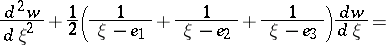(*)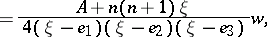whereis natural number and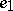,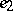,, andare constants, has a solution of one of the following forms: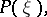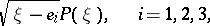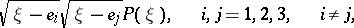where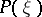is a polynomial with leading coefficient one, then this solution is called a Lamé function of degreeof the first kind and the first, second, third, or fourth form, respectively.

For fixed eventhere are always values of(eigen values) such that there are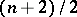Lamé functions of the first form and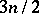of the third form, with polynomialsof degree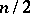and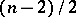, respectively. For fixed oddthere are always values ofsuch that there are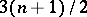Lamé functions of the second form and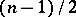of the fourth form, with polynomialsof degreeand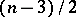, respectively. For a given natural numberthere are altogetherlinearly independent Lamé functions.

Solutions of equation (*) that are linearly independent with the Lamé functions of the first kind and are obtained by means of the Liouville–Ostrogradski formula are called Lamé functions of the second kind.

For references see Lamé equation.

How to Cite This Entry:
Lamé function. Encyclopedia of Mathematics. URL: http://encyclopediaofmath.org/index.php?title=Lam%C3%A9_function&oldid=47572
This article was adapted from an original article by N.Kh. Rozov (originator), which appeared in Encyclopedia of Mathematics - ISBN 1402006098. See original article